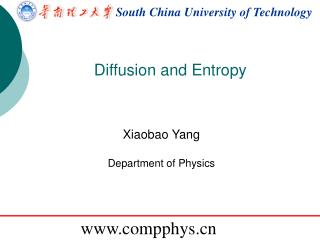Download PresentationSouth China University of TechnologySouth China University of Technology - PowerPoint PPT Presentation

Download PresentationSouth China University of Technology
An Image/Link below is provided (as is) to download presentation

Download Policy: Content on the Website is provided to you AS IS for your information and personal use and may not be sold / licensed / shared on other websites without getting consent from its author. While downloading, if for some reason you are not able to download a presentation, the publisher may have deleted the file from their server.

- - - - - - - - - - - - - - - - - - - - - - - - - - - E N D - - - - - - - - - - - - - - - - - - - - - - - - - - -
Presentation Transcript

1. South China University of Technology Diffusion and Entropy Xiaobao Yang Department of Physics www.compphys.cn

2. 1-D and 2-D random walks • re=zeros(M,N); • re=zeros(M*2,N); • for ii=1:N-1 • for jj=1:M • re(jj,ii+1)=re(jj,ii)+sign(rand-0.5); • if rand <0.5 • re(jj*2-1,ii+1)=re(jj*2-1,ii)+sign(rand-0.5); • re(jj*2,ii+1)=re(jj*2,ii); • else • re(jj*2,ii+1)=re(jj*2,ii)+sign(rand-0.5); • re(jj*2-1,ii+1)=re(jj*2-1,ii); • end • end • end plot(sum(re.^2,1)/M)

3. Random Walks and Diffusion P(i,j,k,n) is the probability to find the particle at the site (i,j,k) at time n. where That is the diffusion Eq:

4. Random Walks and Diffusion is similar to the wave Eq.: The numerical approach adopted for wave Eq. could be applied here too. NB: choose Δt, Δx properly to avoid numerical instability.

5. Simulation of one dimensional Initial δ-function distribution: Unexpected zero’s? Solution: a) average neighbor sites, b) spread initial density profile to many grid sites. Typically, the diffusion could be described as:

6. Simulation of cream in coffee

7. Diffusion, Entropy, and the Arrow of Time Statistical definition of entropy:

8. Diffusion, Entropy, and the Arrow of Time ln64=4.159 rwtd.m

9. Simulation of Diffusion N=10000; %steps % M=200; %number of particles re=zeros(M*2,N)+4; % position storage %start at the center for ii=1:N-1 re(:,ii+1)=re(:,ii); movesite=ceil(rand*M); if rand <0.5 tmp=re(movesite*2-1,ii)+sign(rand-0.5); re(movesite*2-1,ii+1)=tmp-(tmp>8)*2+(tmp<1)*2; re(movesite*2,ii+1)=re(movesite*2,ii); else tmp=re(movesite*2,ii)+sign(rand-0.5); re(movesite*2,ii+1)=tmp-(tmp>8)*2+(tmp<1)*2; re(movesite*2-1,ii+1)=re(movesite*2-1,ii); end end

10. Calculation of entropy re(ii,jj) in [1,8] for jj=1:N ts(jj)=0; s=zeros(8,8); for ii=1:M s(re(ii*2-1,jj),re(ii*2,jj))=s(re(ii*2-1,jj),re(ii*2,jj))+1; end for ii=1:64 if s(ii)~=0 ts(jj)=ts(jj)-s(ii)/M*log(s(ii)/M); end end end plot(ts) S is a 8x8 matrix and equal to 1x64 vetor

11. Parrondo‘s Paradox Engineers know that two unstable systems, if combined in the right way, can paradoxically become stable. But can two losing gambling games be set up such that, when they are played one after the other, they becoming winning? The answer is yes. This is a striking new result in game theory called Parrondo’s paradox, after its discoverer, Juan Parrondo. "Losing strategies can win by Parrondo's paradox", G. P. Harmer and D. Abbott, Nature 402 (1999), 864

12. Losing strategies can win by Parrondo’s paradox

13. Simulation of Game A • e=0.005; • p1=0.5-e; • N=10000; • C=zeros(N,1); • C(1)=N; • for ii=1:N-1 • C(ii+1)=C(ii)+2*(rand<p1)-1; • end • plot(C,'o-')

14. Simulation of Game B • M=3; e=0.005; • p2=0.1-e; p3=0.75-e; • N=10000; • C=zeros(N,1); • C(1)=N; • for ii=1:N-1 • if mod(C(ii),M)==0 • C(ii+1)=C(ii)+2*(rand<p2)-1; • else • C(ii+1)=C(ii)+2*(rand<p3)-1; • end • end

15. Random combination of A and B M=3; e=0.005; p1=0.5-e; p2=0.1-e; p3=0.75-e; N=10000; C=zeros(N,1); C(1)=N; • for ii=1:N-1 • gamekind=rand; • if gamekind<0.5 %game A • C(ii+1)=C(ii)+2*(rand<p1)-1; • end • if gamekind>0.5 %game B • if mod(C(ii),M)==0 • C(ii+1)=C(ii)+2*(rand<p2)-1; • else • C(ii+1)=C(ii)+2*(rand<p3)-1; • end • end • end

16. Brownian ratchet The Brownian ratchet or Feynman-Smoluchowski ratchet is a thought experiment about an apparent perpetual motion machine first analyzed in 1912 by Polish physicist Marian Smoluchowskiand popularized by American Nobel laureate physicist Richard Feynman in a physics lecture at the California Institute of Technology on May 11, 1962, and in his text The Feynman Lectures on Physics as an illustration of the laws of thermodynamics.

17. Why the machine fails? How it works: a gear known as a ratchet that rotates freely in one direction but is prevented from rotating in the opposite direction by a pawl; small enough that the impulse from a single molecular collision can turn the paddle; net effect of many such random collisions should be sufficient for the ratchet to rotate continuously in that direction. It is impossible for any device that operates on a cycle to receive heat from a single reservoir and produce a net amount of work. Feynman demonstrated that if the entire device is at the same temperature, the ratchet will not rotate continuously in one direction but will move randomly back and forth, and therefore will not produce any useful work. The reason is that the pawl, since it is at the same temperature as the paddle, will also undergo Brownian motion, "bouncing" up and down. It therefore will intermittently fail by allowing a ratchet tooth to slip backward under the pawl while it is up.

18. Self-Avoiding Walks A typical polymer in solution may be described by SAW. Keep all possible SAW configurations equivalent. To generate 4th steps if a new possible way is chosen when “self-avoiding” • There are 3 possible ways. (only has 2/3 the probability as in b) • There are only 2 possible ways . How to correct the problem? • Discard the whole RW when restriction condition occurred. CPU time ! • “enumeration” instead of “simulation”.

19. saw.m jj=1:sx(1) newtmp=[x(jj,1:2:end)' x(jj,2:2:end)']; % find all the used sites in the same link! tmp=x(jj,end-1:end)+[0 1]; if length(intersect(tmp,newtmp,'rows'))==0; allx=[allx x(jj,:) tmp]; end

20. Self-Avoiding Walks Log-log scale Average of 106 walks Average of 1000 walks ν=1/2: simple random walk ν=3/4: 2D SAW ν~3/5: 3D SAW

21. Random walk in hex- andtri- lattice O (0.00,0.00) A (0.00,1.00) B (-0.87 1.50) C ( 0.87 1.50) T=

22. Homework Simulation of the Parrondo‘s Paradox. Sending to 17273799@qq.com when ready For lecture notes, refer to http://www.compphys.cn/~xbyang/